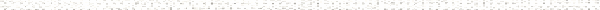A Precalculus Outline

 Chapter 11: Applications of TrigonometryObjective: Examples of Sinusoidal ModelsObjective: Graphs of the Trigonometric Functions (Section 10.5)Objective: Solving Triangles using The Law of SinesObjective: Applications involving Law of SinesObjective: The Ambiguous CaseObjective: Application with the Ambiguous CaseObjective: Finding the Area of a triangle using SineObjective: Degree Measure (Section 10.1)Objective: Solving equations involving the trigonometric functions (Sections 10.6, 10.7)Objective: Solving Triangles using The Law of CosinesObjective: Applications to Law of CosinesObjective: Finding the Area of a Triangle using Heron's FormulaObjective: Degree Measure (Section 10.1)Objective: Solving equations involving the trigonometric functions (Sections 10.6, 10.7)Objective: Introduction to Polar CoordinatesObjective: Converting points between rectangular and polar coordinatesObjective: Convert Polar Equations to Rectangular EquationsObjective: Convert Rectangular Equations to Polar EquationsObjective: The Unit Circle: Cosine and Sine (Section 10.2)Objective: Graphing Polar EquationsObjective: Graphs of the Trigonometric Functions (Section 10.5)Objective: Polar Coordinates (Section 11.4)Objective: General Form of a ConicObjective: Conics (Chapter 7)Objective: Writing complex numbers in polar formObjective: Finding products and quotients of complex numbers in polar formObjective: Finding powers of complex numbers written in polar form (DeMoivre's Theorem)Objective: Finding the roots of a complex numberObjective: Complex Zeros and The Fundamental Theorem of Algebra (Section 3.4)Objective: Polar Coordinates (Section 11.4)Objective: Introduction to VectorsObjective: Representing Vectors Geometrically and AlgebraicallyObjective: Polar Coordinates (Section 11.4)Objective: Bearing Applications (Sections 11.2 and 11.3)Objective: Finding the Dot Product of two vectorsObjective: Finding the angle between two vectorsObjective: Deriving the formula for the angle between two vectorsObjective: Vectors (Section 11.8)Objective: The Inverse Trigonometric Functions (Section 10.6)Objective: Trigonometric Equations and Inequalities (Section 10.7)Objective: Graphing a system of parametric equationsObjective: Converting a system of parametric equations to rectangular formObjective: Systems of Non-Linear Equations and Inequalities (Section 8.7)Objective: Conics (Chapter 7)